# Proof

## Learn how to develop an argument, justification or proof using mathematical language.

### Curriculum

The English national curriculum for mathematics aims to ensure that all pupils reason mathematically by following a line of enquiry, conjecturing relationships and generalisations, and developing an argument, justification or proof using mathematical language.

### Proof

A mathematical proof is a sequence of statements that follow on logically from each other that shows that something is always true. Presenting many cases in which the statement holds is not enough for a proof, which must demonstrate that the statement is true in all possible cases.### Lesson Starter

Introduce the notion of proof with this challenge involving odd numbers - then when the students are convinced it is impossible show them the trick!### Congruent Triangles

Test your understanding of the criteria for congruence of triangles with this self-marking quiz.### Four Colour Theorem

Show that no more than four colours are required to colour the regions of the map or pattern so that no two adjacent regions have the same colour.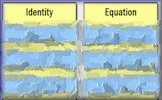### Identity, Equation or Formula?

Arrange the given statements in groups to show whether they are identities, equations or formulae.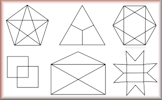### Line Drawings

Six line drawings that may or may not be able to be traced without lifting the pencil or going over any line twice.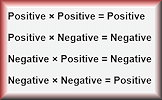### Mix and Math

Determine the nature of adding, subtracting and multiplying numbers with specific properties.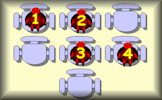### Not Too Close

The students numbered 1 to 8 should sit on the chairs so that no two consecutively numbered students sit next to each other.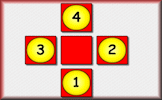### Plus

A number arranging puzzle with seven levels of challenge.### Proof of Circle Theorems

Arrange the stages of the proofs for the standard circle theorems in the correct order.### Satisfaction

This is quite a challenging number grouping puzzle requiring a knowledge of prime, square and triangular numbers.### True or False?

Arrange the given statements in groups to show whether they are always true, sometimes true or false.

### Exam-style Question

State whether each of the following statements is true or false. Give reasons for your answers.

### Exam-style Question

One is added to the product of two consecutive positive even numbers. Show that the result is a square number.

### Exam-style Question

(a) Give a reason why 0 is an even number.

### Exam-style Question

Betsy thinks that $$(3x)^2$$ is always greater than or equal to $$3x$$.

### Exam-style Question

Given that $$n$$ can be any integer such that $$n \gt 1$$, prove that $$n^2 + 3n$$ is even.

### Exam-style Question

Use algebra to prove that $$0.3\dot1\dot8 \times 0.\dot8$$ is equal to $$\frac{28}{99}$$.

### Exam-style Question

The diagram shows a quadrilateral ABCD in which angle DAB equals angle CDA and AB = CD.

### Exam-style Question

m and n are positive whole numbers with m > n

### Exam-style Question

(a) Prove that the recurring decimal $$0.\dot2 \dot1$$ has the value $$\frac{7}{33}$$

### Exam-style Question

(a) Prove that the product of two consecutive whole numbers is always even.

### Exam-style Question

Prove that the expression below is always positive.

### Exam-style Question

Consider two consecutive positive even numbers, $$2n$$ and $$2n + 2$$.

### Exam-style Question

Use mathematical induction to show that:

### Exam-style Question

Consider the sum of the squares of any two consecutive odd integers.

### Exam-style Question

Prove that the integers $$a$$ and $$b$$ cannot both be odd if $$a^2+b^2$$ is exactly divisible by 4.

### Divisible by 11

Can you prove that a three digit number whose first and third digits add up to the value of the second digit must be divisible by eleven?

### Key Eleven

Prove that a four digit number constructed in a certain way will be a multiple of eleven.

### Log Perfection

Determine whether the given statements containing logarithms are true or false

### Two Equals One

What is wrong with the algebraic reasoning that shows that 2 = 1 ?

### Zero Even

Prove that zero is an even number.

### Syllabus

#### 📘

See the requirements for the International Baccalaureate Anaylsis and Approaches Standard Level Mathematics course.

Syllabus Details

### Syllabus

#### 📘

See the requirements for the International Baccalaureate Anaylsis and Approaches Higher Level Mathematics course.

Syllabus Details

### Syllabus

#### 📙

See the content for Mathematics AS and A level as published by the UK's Department for Education.

Syllabus Details

### Tip

#### 💡

If $$n$$ is a whole number then $$2n$$ is an even number.

If $$n$$ is a whole number then $$2n + 1$$ is an odd number.

### Tip

#### 💡

positive × positive = positive
positive × negative = negative
negative × positive = negative
negative × negative = positive

### Tip

#### 💡

even × even = even
even × odd = even
odd × even = even
odd × odd = odd

### Tip

#### 💡

To prove something is not true you only need to find one counterexample.

### Tip

#### 💡

To prove an identity is true show that the left-hand side (LHS) is equal to the right-hand-side (RHS).

### Tip

#### 💡

For 'proof by exhaustion' you may need to understand a topic called:

Combinatorics.

### Tip

#### 💡

Finally here are three letters to conclude your proof:
QED is an initialism of the Latin phrase quod erat demonstrandum, meaning "which was to be demonstrated".

### Teaching Notes:

Many Transum activities have notes for teachers suggesting teaching methods and highlighting common misconceptions. There are also solutions to puzzles, exercises and activities available on the web pages when you are signed in to your Transum subscription account. If you do not yet have an account and you are a teacher, tutor or parent you can apply for one by completing the form on the Sign Up page.